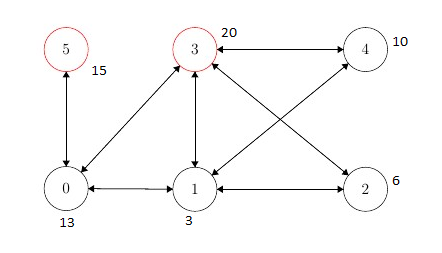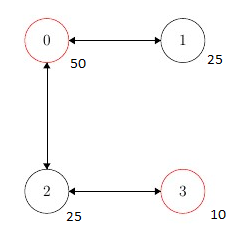Friends
(Main file name: friend.pl)

A social network consists of n people numbered 0, ..., n-1. Some people in the network will become friends. If x is a friend of y, then y is a friend of x.

The people are added to the network in n stages, which are also numbered from 0 to n-1. Person i is added in stage i. In stage 0, person 0 is added as the only person of the network. In each of the next stages, a person is added to the network by a host, who may be any person already in the network. At stage i(0<i<n ), the host for that stage can add the incoming person into the network by one of the following three protocols:

• IAmYourFriend makes person i a friend of the host only. (Represented by protocol 0)
• MyFriendsAreYourFriends makes person i a friend of all the current friends of the host. This does not make person i a friend of the host. (Represented by protocol 1)
• WeAreYourFriends makes person i a friend of the host, as well as a friend of all the current friends of the host (Combines IAmYourFriend and MyFriendsAreYourFriends) (Represented by protocol 2)
After we build the network we would like to pick a sample for a survey, that is, choose a group of people from the network. Since friends usually have similar interests, the sample should not include any pair of people who are friends with each other. Each person has a survey confidence, expressed as a positive integer, and we would like to find a sample with the maximum total confidence.

Input Formats

An input file for LP systems contains the following facts:
• One fact of the form people(N), which specifies the number of  people in the network.
• For each I in 0..N, there is a fact of the form confidence(I,C), which gives the confidence value C of person I.
• For each I in 1..N-1, there is a fact of the form host_protocol(I,H,P), which gives the host H and the protocol P of the stage I . Note that there is no stage 0, so there is no fact host_protocol(0,0,H).

Output format

The output should contain exactly one fact of the form max_confidence(K), where K is the largest possible confidence for any sample in the network.

Examples

 Input Diagram Output people(6). host_protocol(1,0,0). host_protocol(2,1,0). host_protocol(3,1,2). host_protocol(4,2,1). host_protocol(5,0,0). confidence(0,13). confidence(1,3). confidence(2,6). confidence(3,20). confidence(4,10). confidence(5,15).max_confidence(35). people(4). host_protocol(1,0,0). host_protocol(2,1,1). host_protocol(3,2,0). confidence(0,50). confidence(1,25). confidence(2,25). confidence(3,10).max_confidence(60). people(10). host_protocol(1,0,0). host_protocol(2,1,0). host_protocol(3,2,2). host_protocol(4,3,1). host_protocol(5,2,0). host_protocol(6,5,2). host_protocol(7,3,0). host_protocol(8,7,0). host_protocol(9,8,1). confidence(0,20). confidence(1,10). confidence(2,5). confidence(3,40). confidence(4,20). confidence(5,10). confidence(6,15). confidence(7,75). confidence(8,35). confidence(9,45).max_confidence(155).